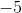Example Questions

1 2 3 5 Next →

Example Question #3115 : Act Math

Solve for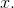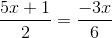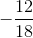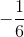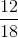Explanation:

To solve these types of problems, you need to cross multiply the fractions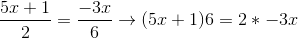.

Then, all we need to do is distribute the, combine like terms, and solve the algebraic equation.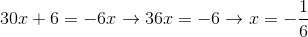.

Example Question #3116 : Act Math

Solve for: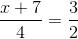Explanation:

Cross-multiply: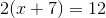Distribute: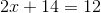Solve for x: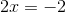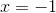Example Question #3117 : Act Math

Solve for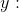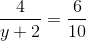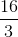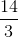Explanation:

Solve this question by cross-multiplying: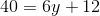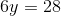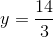Example Question #3241 : Sat Mathematics

If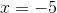, what is the value of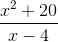?